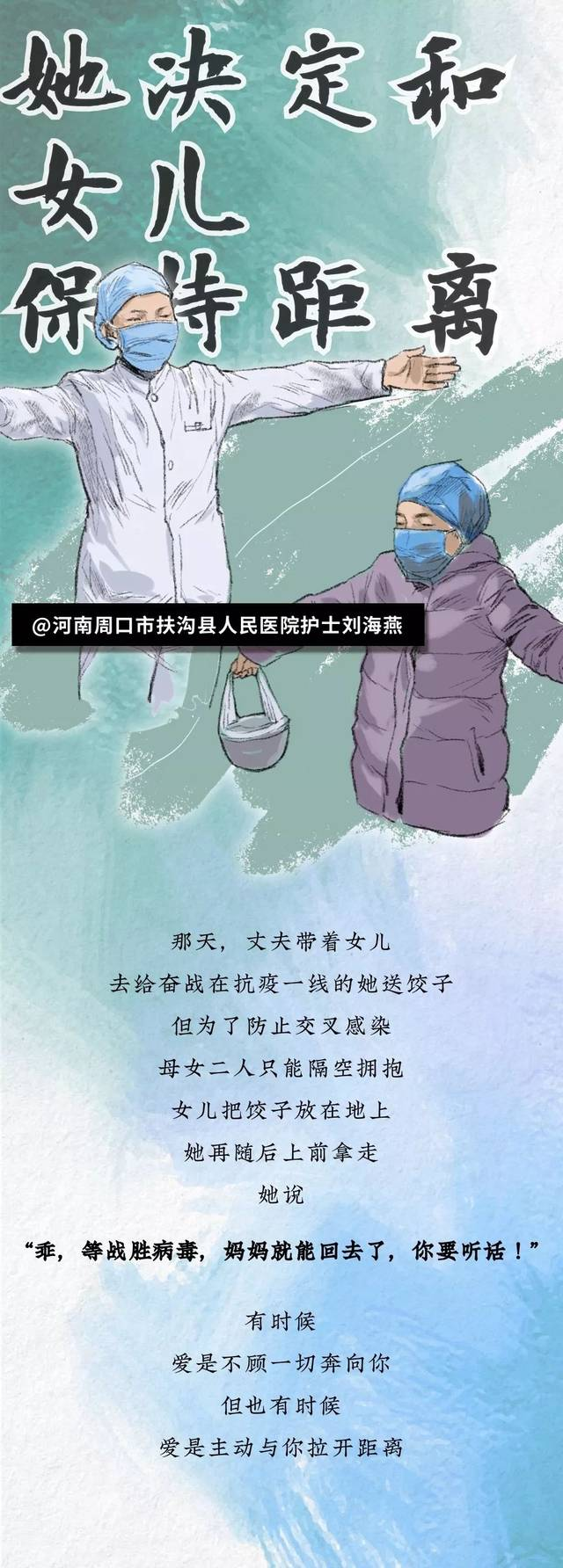1+1+1+1+1+1+1+1+1=？_手机搜狐网

## 1+1+1+1+1+1+1+1+1=？……

1+1+1+1+1+1+1+1+1 = ？

1、疫情中的我们，如何面对自己？

2、疫情中的我们，如何面对工作和事业？

“想尽办法活下去。只要挺过去，迎接我们的就是新的春天。”他说。

3、疫情中的我们，如何面对未来？